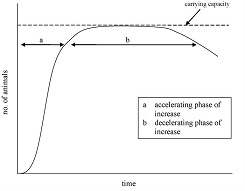Need Assistance? 01384 442752 (UK)

# StatisticsStatistics are important to every sub discipline of applied science.

Statistics (singular) is the science of data analysis. That is, statistics is concerned with scientific methods for collecting data, organising, summarising, presenting, and analysing sample data, as well as drawing valid conclusions and making reasonable decisions based on such analysis.

Statistics are an integral part of the scientific method. There is need of statistical data in every walk of life. No field of study is complete without the supporting quantitative information about that field. Study material in Economics, Commerce, Accountancy, Geography, Physics, Chemistry, Biology etc., are all flooded with quantitative information. No government department can function well without the support of statistical data.

Statistics involves using a variety of different methods and rules for organising and interpreting observations.

Terminology
Statistics has it'sd own language; and if you want to understand statistical data better; it is useful to understand the words that are used:
• Parameter any characteristic of the population
• Statistic any characteristic of a sample
• Data are measurements or observations
• Datum is a single measurement or observation

Characteristics can be expressed:

• Numerically (ie. quantitative or variable); or
• Non-numerically  (ie. qualitative or categorical or attribute)

Numerical Data can be:

• Discrete, with integer based counting (eg. How many things…?, Yes/No converted to numbers (surveys))
• Continuous, with decimal based counting (eg. How tall are you? What are the dimensions of the subject?)

What exactly does statistics measure?
• Constructs - which are theoretical concepts (eg. intelligence, learning, memory)
• Variables - which is a characteristic or condition that changes or has different values for different individuals/objects/programs
• Constants - values that do not change, it remains the same for all individuals/objects/programs

Statistical Distribution Measures are:
• Location or central tendency (mean, median, mode, midrange, quartiles, mid-hinge)
• Shape (continuous or discrete distributions)
• Scatter, spread or dispersion (range, location or central tendency shape with continuous or discrete distributions)

[11/08/2022 18:38:16]